Goseeko blog# What is the State Transition matrix and its properties?

The State transition matrix is that matrix whose product with the state vector at initial time gives the value of variable x for time t. The state transition matrix is helpful for finding controllability, general solution, observability and stability of the LTI system.

## State Transition Matrix and its Properties:

As we know the output equation is given as

y(t)=Cx(t)+Du(t)

x(t)=Ax(t)+Bu(t)

d(x)/dt -Ax(t)=Bu(t)

Taking Laplace transform of above equation we have

SX(s)-X(0)-AX(s)=BU(s)

SX(s)-AX(s)=BU(s)+X(0)

[SI-A]X(s)=X(0)+BU(s)

X(s)=[SI-A]-1[X(0)+BU(s)]

X(s)=[SI-A]-1X(0)+[SI-A]-1BU(s)                                       (a)

This is solution of state differential equation

L-1X(s)= L-1{[SI-A]-1X(0)+[SI-A]-1BU(s)}

x(t)= [SI-A]-1x(0)+ [SI-A]-1Bu(t)                                        (b)

From above x(t) we can find output equation by replacing x(t) in output equation by its value from above equation(b)

For the given system below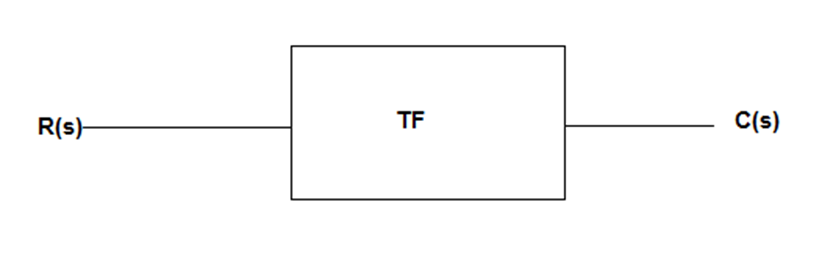The transfer function of above system is given as

TF=c(t)/r(t)

This c(t) is the output of the present system, which is not equal to above y(t), as their initial conditions are not considered.

If initial conditions are zero than both y(t) and c(t) will be equal to

L-1[SI-A]-1=φ(t)…….. state transition matrix

Φ(s)=[SI-A]-1

x(t)=φ(t)x(0)+L-1[φ(s)* BU(s)]

When the input is zero i.e. u(t) =0 the State transition matrix satisfies the solution of the state equation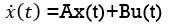As u(t)=0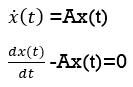Solution to above equation is

y(t)=Ke-Pt+e-Pt∫ ePt Q d(t)

But [dx(t)/dt] -Ax(t)=0

Hence above equation becomes

X(t)=AeAt

Substitute t=0

x(0)=ke0

x(0)=k

x(t)=x(0) e At                (zero input response)

The above equation is the Zero Input Response of the system.

## Properties

From above equation when u(t)=0

x(t)=φ(t) x(0)

and from zero input response we have

φ(t)=eAt

Property 1:

φ(0)= [I]

Property 2:

Φ-1(t)= [φ(t)]-1=e-At=eA(-t)

Φ-1(t)= Φ(-t)

Property 3:

ΦK(t)= [Φ(t)]K

ΦK(t)=[eAt]K=eA(tK)

Then ΦK(t)= Φ(Kt)

Property 4:

Φ(t1+t2)=eA(t1+t2)

=e(At1+At2)=eAt1 * eAt2

Φ(t1+t2)= Φ(t1)Φ(t2)

Property 5:

Φ(t2-t1) * φ(t1-t0)=e A(t2-t1) * e A(t1-t0)

Φ(t2-t1) * φ(t1-t0)= Φ(t2-t0)

Example

1. If matrix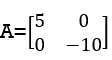Find the state transition matrix?

Solution: The state transition matrix is given by L-1[SI-A]-1=φ(t)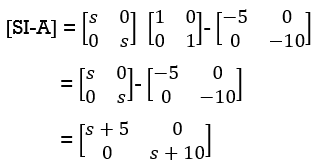Taking inverse Laplace of above, we get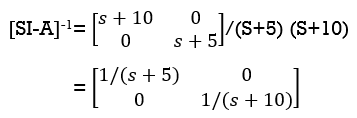Hence φ(t)=L-1[SI-A]-1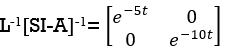2) Find φ(t) if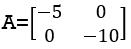Solution: As we already know that L-1[SI-A]-1=φ(t)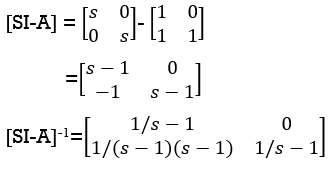Hence φ(t)=L-1[SI-A]-1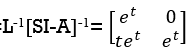Interested in learning about similar topics? Here are a few hand-picked blogs for you!